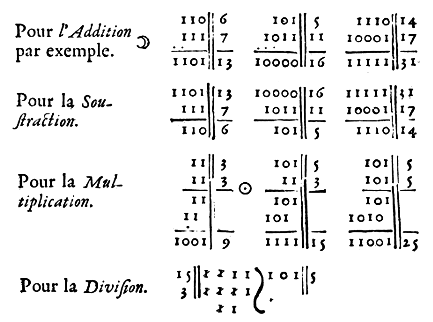## Binary numbers are a base 2 numeral system.

A binary number is a number expressed in the base-2 numeral system, which uses only two symbols: 0 and 1. Each digit is referred to as a bit. Because of its straightforward implementation in digital electronic circuitry using logic gates, the binary system is used by almost all modern computers and computer-based devices.

• Bit: The smallest unit in computers. It is either a 1 or a 0.
• Nibble: Half a byte, or 4 bits.
• Byte: 8 bits together form one byte, a number from 0 to 255. Bits in a byte are numbered starting from the right at 0.
• Short: Two bytes put together are 16 bits, forming a number from 0 to 65535. The low byte is the rightmost eight bits.
• Big Endian: Stores data big-end first. When looking at multiple bytes, the first byte (lowest address) is the biggest.
• Little Endian: Stores data little-end first. When looking at multiple bytes, the first byte is smallest.

### Conversion

Break down the binary value in chunks of 4, multiply each 1 by its equivalent value, either 8, 4, 2 or 1. Add the resulting numbers together to get the result. For example, the value 1100, or (8*1 + 4*1), is equal to C(decimal 12).

`1101 0101(D5)`
11010101Binary
1286432168421Values
128641641Result: 213

### Binary Arithmetic

In the first column, divide the first number by 2 by removing the last bit, until 1 is reached. In the second column, multiply by 2 by adding an extra bit of 0. The answer is found by adding the numbers in the second column with odd numbers in the first column. A binary number ending with 1 is odd.

3519
`100011`` 10011`
` 10001`` 100110`
` 1000`` 1001100`
` 100`` 10011000`
` 10`` 100110000`
` 1``1001100000`
`1010011001`

This example multiplies 35 by 19, to arrive at a result of 665. The result `1010011001` can be deconstucted as:

`10 1001 1001 = 1 + 8 + 16 + 128 + 512 = 665`

Binary numbers can be multiplied and divided by multiples of 2, by rotating one bit left to multiply by 2, or one bit right to divide by 2.

 22 44 `ROL` 11 `ROR` 0 0 0 1 0 1 1 0 0 0 1 0 1 1 0 0 0 0 0 0 1 0 1 1

AND, or "both", sets individual bits to 0. AND is useful for masking bits, for example, to mask the high order bits of a value AND with \$0F: \$36 AND \$0F = \$06. ORA(OR), or "either one or both", sets individual bits to 1. OR is useful for setting a particular bit, for example, \$80 OR \$08 = \$88. EOR(XOR), or "one or the other but not both", inverts individual bits.

 input output AND ORA EOR 0 1 0 1 1 0 0 0 0 0 1 1 1 1 0 1 0 0 1 1

To activate the 1st, 2nd and 4th bits:

 0 0 0 0 1 0 1 1
```unsigned char num = 0;
num |= (1 << 0);
num |= (1 << 1);
num |= (1 << 3);
```

To deactivate the 1st, 4th and 6th bits:

 1 1 0 1 0 1 1 0
```unsigned char num = 255;
num &= ~(1 << 0);
num &= ~(1 << 3);
num &= ~(1 << 5);
```

`(num >> bit) & 1;`

If Bit 7 is not set (as in the first example) the representation of signed and unsigned numbers is the same. However, when Bit 7 is set, the number is always negative. For this reason Bit 7 is sometimes called the sign bit.

 Binary Unsigned Signed 0010 0011 35 35 1010 0011 163 -93 1111 1111 255 -1 1000 0000 128 -128

A fixed-point number is a number that has a fixed number of digits after the decimal point. If, for example, we use 8 bits to store a number with decimal points, we could decide to store it this way. The high and low nibbles have the same resolution.

Integer PartDecimal Points
000000000.0
001110103.a
010001014.5

To turn a regular integer into fixed point, shift left by the number of fractional bits(width << bits), and to turn a fixed point into integer, shift right by the number of fractional bits(width >> bits).

To multiply, you do the multiply, and then you shift right by the number of fractional bits.

`(3.8 * 2.0) >> 8`

To divide, you first shift the numerator left by the number of fractional bits, then you do the division.

`(3.8 << 8) / 2.0`